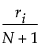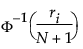Publication date: 04/12/2021

## Normal Quantile Plot

The empirical cumulative probability for each value is computed as follows:where ri is the rank of the ith observation, and N is the number of nonmissing (and nonexcluded) observations.

The normal quantile values are computed as follows:where Φ is the cumulative probability distribution function for the normal distribution.

These normal quantile values are Van Der Waerden approximations to the order statistics that are expected for the normal distribution.

Want more information? Have questions? Get answers in the JMP User Community (community.jmp.com).